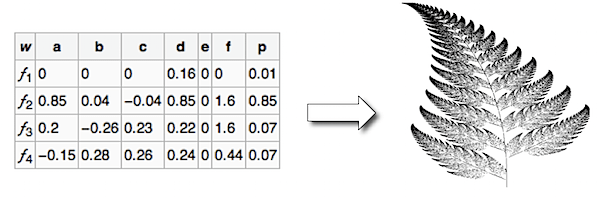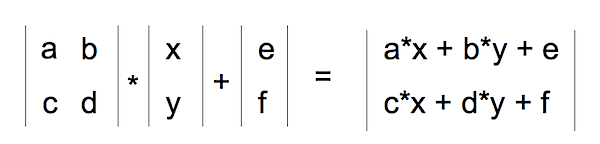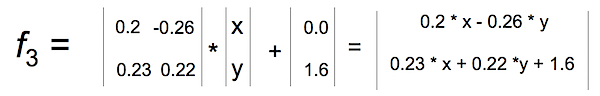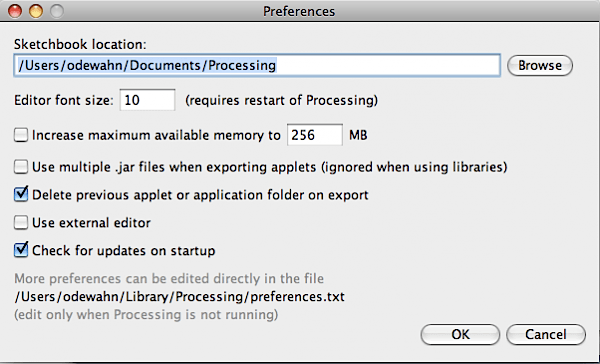A fractal, according to Wikipedia, is a “rough or fragmented geometric shape that can be split into parts, each of which is (at least approximately) a reduced-size copy of the whole.” One of the cool things about fractals is that they use really simple processes to create really complex, natural looking objects, like plants, clouds, flames, and so forth. This Codebox will show you how to create a fractal menagerie for viewing a few different styles of fractals, like this:

Along the way, we’ll continue to expand on the object-oriented programming (OOP) concepts introduced in Chapter 9 of Getting Started with Processing, as well as show how to use the great controlP5 library to create familiar GUI controls used in other languages.

IFS Fractals

The Iterated Function System, or IFS, is one of the simplest techniques for creating natural-looking fractals. The IFS consists of a handful of transformations (more on these in a second) that describe how to change one point into another. The Barnsley Fern, which looks like a Black Spleenwort (a kind of fern), is the classic example of an IFS fractal, but there are many varieties. Larry Riddle has a nice breakdown of others on his IFS systems page.

The technique works like this. Starting from a single point, usually (0,0), you randomly apply one of the transformations that make up the IFS system. Then, once you have the new, transformed point, you plot it on the screen, which in Processing, we do with the set() command. Then, you repeat the process again starting from the point you just computed.

After a few hundred iterations, you’ll begin to see a ghostly outline of a fern. After 100,000 iterations, you’ll see a detailed image that looks almost exactly like a natural object. The following image shows the IFS system for the Barnsley fern, as well as the image that results when you apply the process over and over.Let’s unpack the details a bit more. A transformation is a formula that…well, transforms one point into another point. Just like the translate, rotate, and scale transformations described on page 104 of Getting Started with Processing, the transformations in the IFS system map a point to a new location by applying a transformation matrix, which is a shorthand way of representing a set of formulas.

The table that defines the IFS system provides the values used in the matrices. Columns a,b,d,d,e, and f represent the various elements in a matrix. (I’ll talk about column p in a second.) The next figure shows how to translate a row in an IFS system into a matrix:As an example, here’s the 3rd transformation matrix for the Barnsley Fern that was shown earlier:The final column in the IFS matrix, p, represents the frequency with which the transformation is selected. So, for the Barnsley fern, transformation f1 is selected 1% of the time, f2 is selected 85% of the time, and f3 and f4 are each selected 7% of the time. If this is a bit confusing, think of as though you filled up a bag with 100 marbles, each labeled each with one of these functions. You’d have 1 marble for f4, 85 for f2, 7 for f3, and 7 for f4. At each iteration, you’d randomly grab a marble from the bag, look at its label, apply the corresponding transformation, and then drop the marble back into the bag.

controlP5

Now that you understand the basic idea of the IFS fractals, let’s take a quick look at controlP5, a code library by Andreas Schlegel for creating familiar user input controls, like these:We’re going to use the “knob” control to allow the user to set the number of iterations used to create the drawing, and the “radio” control to select which IFS system to display.

Before we can use the library in our sketch, we have to get it installed. The first step is to make sure you have a directory called “libraries” in your Sketchbook directory. You can find the Sketchbook directory by opening the Processing’s Preferences window. You’ll see the directory name at the top:After you’ve located the main directory, use a tool like Window’s Explorer, Mac’s finder, or UNIX’s trusty terminal, to see if there is a subdirectory called “libraries.” If so, you’re all set. If not, then create the subdirectory (be sure to name it “libraries”).After the library is installed, restart Processing. Now we’re ready to talk a little bit about what the fractal side of the project.

Set up the sketch

Whew! That was a lot of overview material, but now that controlP5 is installed we’re ready to use Processing to implement the IFS system described earlier.
Start Processing, and then paste the following code into the text editor. (As always, you can either highlight all the code in the following box and then hit ctrl_c, or you can click on this link to controlp5_fractals.pde and then use the ctrl+a, ctrl+c combination.) Here’s the codebox:

There’s one thing you’ll probably notice as you go through the example: it contains multiple class definitions at the end of the file. In the last Codebox, Swat and (arraylist) of targets, we put all the classes into separate tabs. But, this isn’t required — it’s totally fine to lump them all into a single file, like I’ve done here. If you want to split them out into separate tabs, just be sure to name the file the same as the class.

Fire up the code and you should see something very similar to the video at the beginning of this post. You can click the radio buttons to select one from among a variety of different IFS fractals, and can also use the knob controller to set the number of iterations.

Discussion

The code defines three classes that serve as the building block for the project:

• The Transform class. This class performs all math involved in the transformation matrix. Most of the code is devoted to simply storing all the variables. The evalX() and evalY() return the new X and Y coordinates for the point you pass in.
• The IFS class. This class implements the IFS data structures. It consists of an ArrayList of Transform classes called tranforms, an ArrayList to represent the frequency distribution of those transforms called histogram (extending our earlier analogy, this is the bag of marbles we’ll use to select the random transform from), and a description. The key methods are: are addTransform(), which adds a new Transform to the transform list; addHistogram(), which adds a new “marble” to the histogram frequency distribution based on the Transform’s p value; and selectRandomTransform(), which is used to select one of the transforms at random based on the frequency distribution specified in the histogram ArrayList.
• The Point class. Holds the x and y coordinate for the points in the fractal.

We use these classes in a variety of ways in the main program. First, in what’s probably the most conspicuous portion of the code, we use IFS and Transform to define the various fractals we want to display. For example, here’s the code to create the Barnsley Fern:

``````
c = new IFS("Classic Barnsley Fern");
c.addTransform( new Transform( 0.0,   0.0,   0.0,  0.16, 0.0, 0.0,  0.01));
c.addTransform( new Transform( 0.85,  0.04, -0.04, 0.85, 0.0, 1.6,  0.85));
c.addTransform( new Transform( 0.2,  -0.26,  0.23, 0.22, 0.0, 1.6,  0.07));
c.addTransform( new Transform(-0.15,  0.28,  0.26, 0.24, 0.0, 0.44, 0.07));
```
```

And, here’s the code to define the Dragon curve:

``````
c = new IFS ("Dragon");
c.addTransform( new Transform(0.5, -0.5, 0.5, 0.5, 0.0,0.0,.50));
``````
``` ```

Next, we set up controlP5 knob and radio button. This requires 2 parts steps. The first occurs in the setup() method, where the two controls are added to the sketch. Adding the knob is fairly straightforward, and requires only one line:

``````
iterationKnob = controlP5.addKnob("iterationKnob", N_MIN, N_MAX, N, 10,10,50);
```
```

The radio button requires just a bit more code because in addition to adding the control itself, we also have to add the items that can be selected. This is done by looping through the ArrayList of IFS patterns and then using description field as the selection item in the radio button. Here's the code:

``````
for (int i=0; i < patterns.size(); i++) {
IFS p = (IFS) patterns.get(i);
}
```
```

The second part of using the controlP5 library is to set up action methods that are triggered when the user interacts with the control. The key is to give the method the same name as the *description* you used when you added the control, not the name of the control's instance variable. For example, when we added the ifsRadio control, we used ifsSelect as the description. This means that we will need a corresponding method called ifsSelect() to receive any clicks users make on the control. This method must also have a parameter that will hold the current value of the control.

So, for the ifsSelect() method, we have a parameter that will tell us the index of the list item that was just selected. We'll then use that index to retrieve the correct IFS pattern and make it the current one being displayed. The following diagram summarizes all the elements in play. (The controlP5 controls all work slightly differently, but the site has great examples of how to use them all.):The final piece of the puzzle is the makeIFS() method, which makes the actual calculations required to create the fractal. Because IFS systems are so elegant, there really isn't very much to say. We simply loop through the number of iterations specified in the variable N (whose value has been set by the iterationKnob() method), pull out a transform from the current IFS system (whose value has been set in the ifsSelect() method), and then add each point into the array of Point objects. We also keep track of the ranges of the X and Y values so that we can scale the image to fill up the drawing area.

More:
See all of the Codebox columns

In the Maker Shed:Getting Started with Processing
Learn computer programming the easy way with Processing, a simple language that lets you use code to create drawings, animation, and interactive graphics. Programming courses usually start with theory, but this book lets you jump right into creative and fun projects. It's ideal for anyone who wants to learn basic programming, and serves as a simple introduction to graphics for people with some programming skills.

## 1 thought on “Codebox: Create a fractal menagerie”

1. Pingback: wildstar platinums